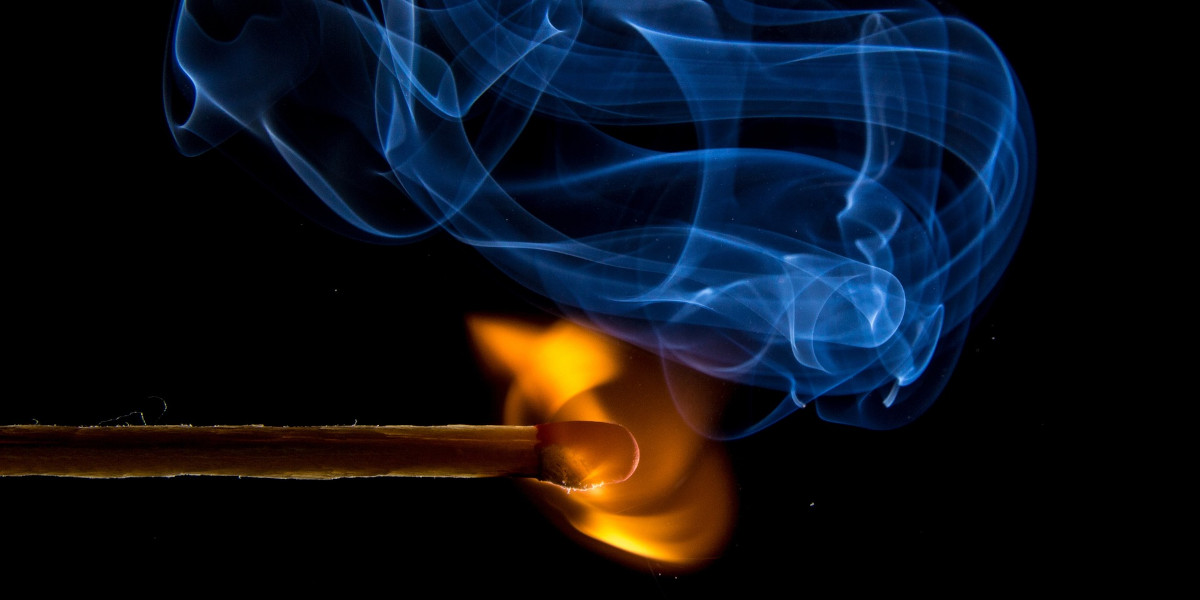Blog

News

## Part 1: What Is Heat? Understanding Temperature | Diabatix

March 03, 2023by
Niels VerdijckUnderstanding heat and temperature is essential in many aspects of our daily lives, from cooking and heating our homes to managing our bodies' temperature. Heat is the transfer of energy from one object to another because of a difference in temperature until they reach thermal equilibrium or the same temperature. Heat is this expressed in Joules, like any form of energy.

As we know, the molecules that make a substance are not stationary. Temperature, therefore, measures the average kinetic energy of individual molecules within an object. The faster the molecules move, the higher the temperature. The internal energy, another important concept in thermodynamics, refers to the total energy of all the molecules within an object.

Temperature is measured in degrees Celsius, Fahrenheit, or Kelvin, with Kelvin being the standard unit in thermodynamics. It is important to note that temperature is not the same as heat. Depending on their masses and specific heat capacities, two objects can have the same temperature but different amounts of heat. Understanding the relationship between heat and temperature is crucial for many fields, including engineering, physics, and meteorology.

When two objects with different temperatures come into contact, heat will flow from the warmer object to the cooler entity. This process involves the transfer of internal energy, causing the temperature of the warmer object to decrease and the temperature of the cooler one to increase. Heat transfer will continue until both objects reach the same temperature, known as thermal equilibrium. At this point, there is no further net heat transfer between them as they have equal temperatures.

When heat is transferred between two objects, the temperature rise or fall depends on the specific heat of the materials involved. Specific heat is expressed in units of Joules per kilogram per degree Celsius (J/kg·°C) and measures how much energy is required to raise the temperature of a unit mass of material by one degree Celsius.

From our definitions early, we know that temperature relates to the average kinetic energy of the molecules. The more molecules an object has - the more mass it has - the more heat has to be transferred to increase temperature.

The specific heat is a material property that expresses how easy it is for a material to increase or decrease in temperature. The lower this value, the less energy is required for this temperature difference (for a fixed amount of mass) and vice versa.

We thus get to the final equation:

$Q = m\:c_\mathrm{p}\:\Delta T$

### Thermodynamics + example use case

Everything that is covered thus far is the basics of thermodynamics. Thermodynamics is the study of processes in which energy is transferred as heat and as work.

Usually, the work is the useful part of this energy transfer, while heat is the nasty by-product - for example, in a lighting fixture. The useful work is that electric energy is converted into light energy. Some of you will have noticed that heat is also produced. This is why you never touch a light bulb after turning it on for a while.

Throughout the remainder of this article series, the light bulb will help explain the covered concepts.

A very important concept within the field of thermodynamics is the conservation of energy. Energy cannot be created out of thin air, nor can it be destroyed. It can pass from one form to another (for example, electrical energy into light energy or electrical energy into heat). This concept is of such importance that it is called the first law of thermodynamics or the law of conservation of energy, which can also be expressed in an equation:

$\Delta E = \Delta E_{\mathrm{kinetic}} + \Delta E_{\mathrm{potential}} + \Delta E_{\mathrm{internal}}=Q-W$

Where $$Q$$ is positive when heat is added to the system and negative when it leaves. $$W$$ represents the work done by the system and it is positive if net work is done by the system. Or in a steady state (which means no changes are allowed), the net work of the system must equal all the heat transfer:

$Q=W$

The next article of this series will discuss heat transfer mechanisms. Be the first to hear about our latest innovations and product releases by following our company page on LinkedIn.

Get started with ColdStream
Discover how our generative design software will help you during every phase of the cooling design process - from optimizing first designs to virtual testing and detailed analysis.